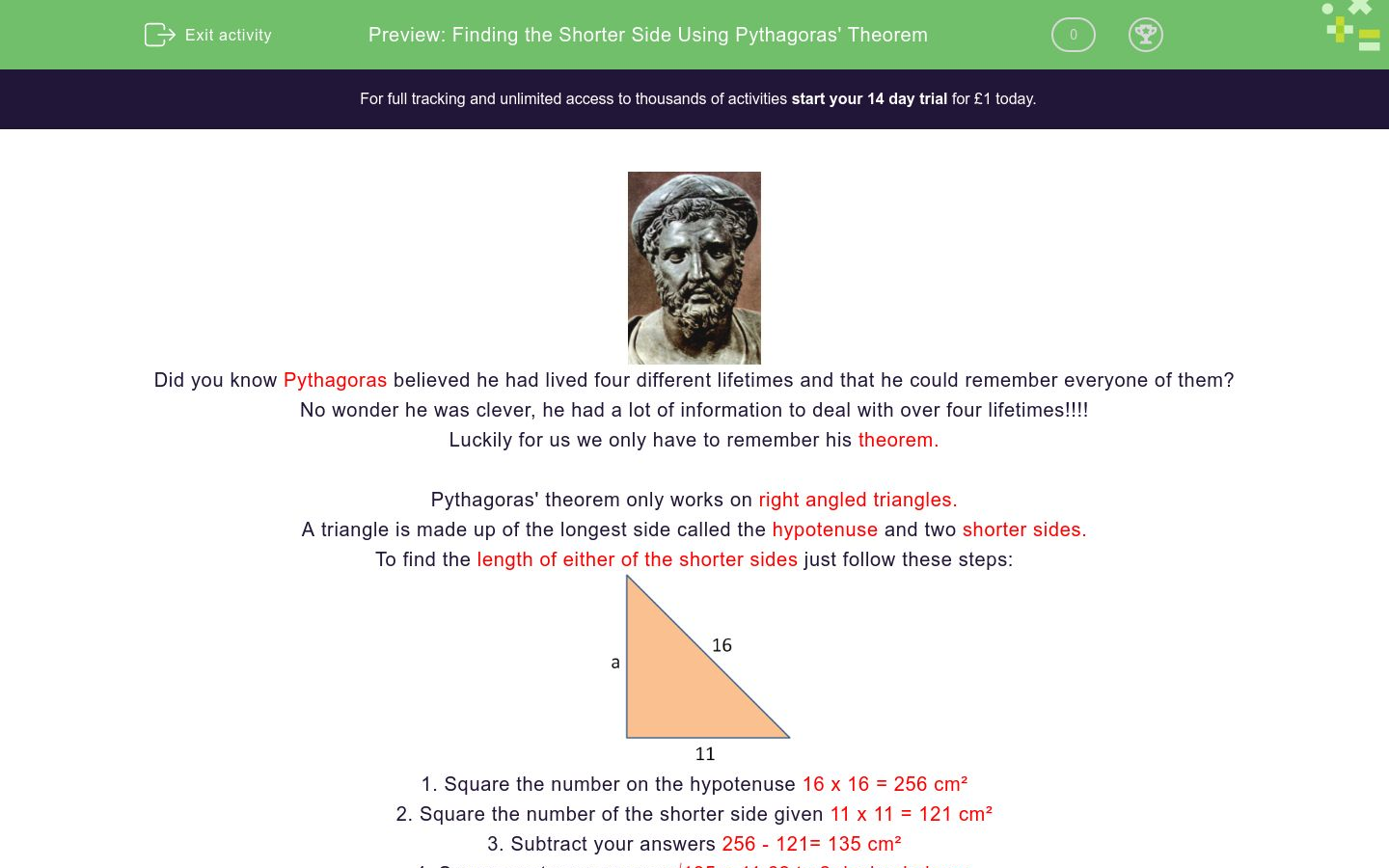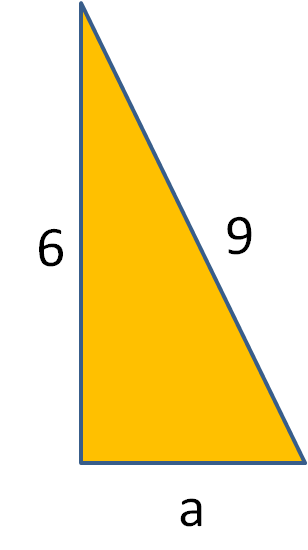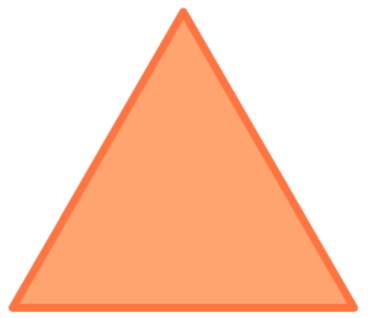# Finding the Shorter Side Using Pythagoras' Theorem

In this worksheet, students will learn how to find the shorter side length of a right angled triangle using Pythagoras' Theorem.Key stage:  KS 4

GCSE Subjects:   Maths

GCSE Boards:   AQA, Eduqas, Pearson Edexcel, OCR

Curriculum topic:   Geometry and Measures, Mensuration

Curriculum subtopic:   Mensuration and Calculation, Triangle Mensuration

Difficulty level:### QUESTION 1 of 10Did you know Pythagoras believed he had lived four different lifetimes and that he could remember everyone of them?

No wonder he was clever, he had a lot of information to deal with over four lifetimes!!!!

Luckily for us we only have to remember his theorem.

Pythagoras' theorem only works on right angled triangles.

A triangle is made up of the longest side called the hypotenuse and two shorter sides.

To find the length of either of the shorter sides just follow these steps:1. Square the number on the hypotenuse 16 x 16 = 256 cm²

2. Square the number of the shorter side given 11 x 11 = 121 cm²

4. Square root your answer √135 = 11.62 to 2 decimal placesAll the s's.

Think Shorter Sides Subtract and square root.

As it is the shorter side we want to find, your answer will never be larger than the length of the hypotenuse.

This is a good way to check your accuracy.  It is always good to check.Find the length of aFind the length of a

7.62 cm

5. 34 cm

6.32 cm

8.45 cmCalculate the length of a.

7.62 cm

5. 34 cm

6.32 cm

8.45 cmWhat is the length of h?What is the length of d?

13.23

13.28

14.56

14.6512.31 cm 16.97 cm 11.31 cm 17.25 cm Length of a6.71 cm 7.61 cm 7.23 cm 6.82 cm Length of a6.71 cm 7.61 cm 7.23 cm 6.82 cm Length of aWhat is the height of this triangle?

Base = 14 cm, slant height = 18 cm

14.54 cm

18. 35 cm

16.58 cm

15.28 cmWhat is the length of half of the base of this triangle?

Height = 12 cm, slant height = 16 cm

• Question 1Find the length of a

5.20
EDDIE SAYS
This is just a case of remembering the fours steps Square 6 x 6 = 36 Square 3 x 3 = 9 Subtract 36 - 9 = 27 Square root = 5.196 (5.20 to 2 d.p) As you can see the answer is shorter than the length the hypotenuse. I wonder how Pythagoras' actually came up with this theorem.
• Question 2Find the length of a

6.32 cm
EDDIE SAYS
Are you remembering the S mantra? Square, Square, Subtract and square root I wonder if Pythagoras used to chant that to himself to remember. 7² - 3² = 40 √40 = 6.32
• Question 3Calculate the length of a.

EDDIE SAYS
What newspaper does a triangle read? The hypotenews. Just checking in that you are awake. There really is only one way to do this calculation. Square, Square, Subtract and square root. 100 - 16 = 84 √84 = 9.17 to 2 d.p
• Question 4What is the length of h?

10.25
EDDIE SAYS
Sometimes when triangles are flipped around it is hard to tell which is the hypotenuse and you may end up using the calculation to find the longest side. If you visualise this with the right angle at the base of the triangle and the triangle pointing up you can see that 11 is clearly the hypotenuse so the missing length is a shorter side. 11² - 4² =√ 105 = 10.25
• Question 5What is the length of d?

13.23
EDDIE SAYS
Are you getting used to this now? It is just a case of practicing the technique. There are so many techniques to remember in maths. Pythagoras' theorem is probably the most famous one. Square, Square, Subtract and Square root. 400 - 225 = √ 175 = 13.23
• Question 612.31 cm 16.97 cm 11.31 cm 17.25 cm Length of a
EDDIE SAYS
Remember to always check that your answer is smaller than the hypotenuse. There really is no other way to do this other than 12² - 4² = 128 cm √ 128 = 11.31 cm
• Question 76.71 cm 7.61 cm 7.23 cm 6.82 cm Length of a
EDDIE SAYS
This theorem works for either of the shorter sides which is great as we wouldn't want to remember a different one for each side would we. 81 - 36 = 45 √45= 6.71 to 2 d.p
• Question 8EDDIE SAYS
It could be tempting to just carry out the calculation using your calculator and write down the answer. Remember in examinations every step of your working must be shown to get marks. So make sure you write each stage down. It doesn't matter which way you do it either. 9 x 9 = 81 7 x 7 = 49 81 - 49 = 32 √32 = 5.66 81 -49 = 32 √32 = 5.66 9² - 7² = √32 = 5.66
• Question 9What is the height of this triangle?

Base = 14 cm, slant height = 18 cm

16.58 cm
EDDIE SAYS
Now Pythagoras always liked us to have to work a little harder. This is not a right angle triangle so how can we apply this his theorem. Easy we just turn it into a right angle triangle, by drawing a line from the point to the base. We have to be a bit careful here as the base is given as 14 cm, we only want half of it which is 7 cm. 18² - 7² = √275 = 16.58 cm We also know this can't be option B as the height cannot be longer that the hypotenuse.
• Question 10What is the length of half of the base of this triangle?

Height = 12 cm, slant height = 16 cm

10.58
EDDIE SAYS
Hopefully this type of thing won't scare you. Draw the line in from the point to the base, which is the height. This forms our right angled triangle. We know that the height is 12 cm. Slant height is 16 cm Situation now familiar? 16² - 12² = √112 = 10.58 cm Top bloke Pythagoras in helping us sort out what looked like a potential problem.
---- OR ----

Sign up for a £1 trial so you can track and measure your child's progress on this activity.

### What is EdPlace?

We're your National Curriculum aligned online education content provider helping each child succeed in English, maths and science from year 1 to GCSE. With an EdPlace account you’ll be able to track and measure progress, helping each child achieve their best. We build confidence and attainment by personalising each child’s learning at a level that suits them.

Get started